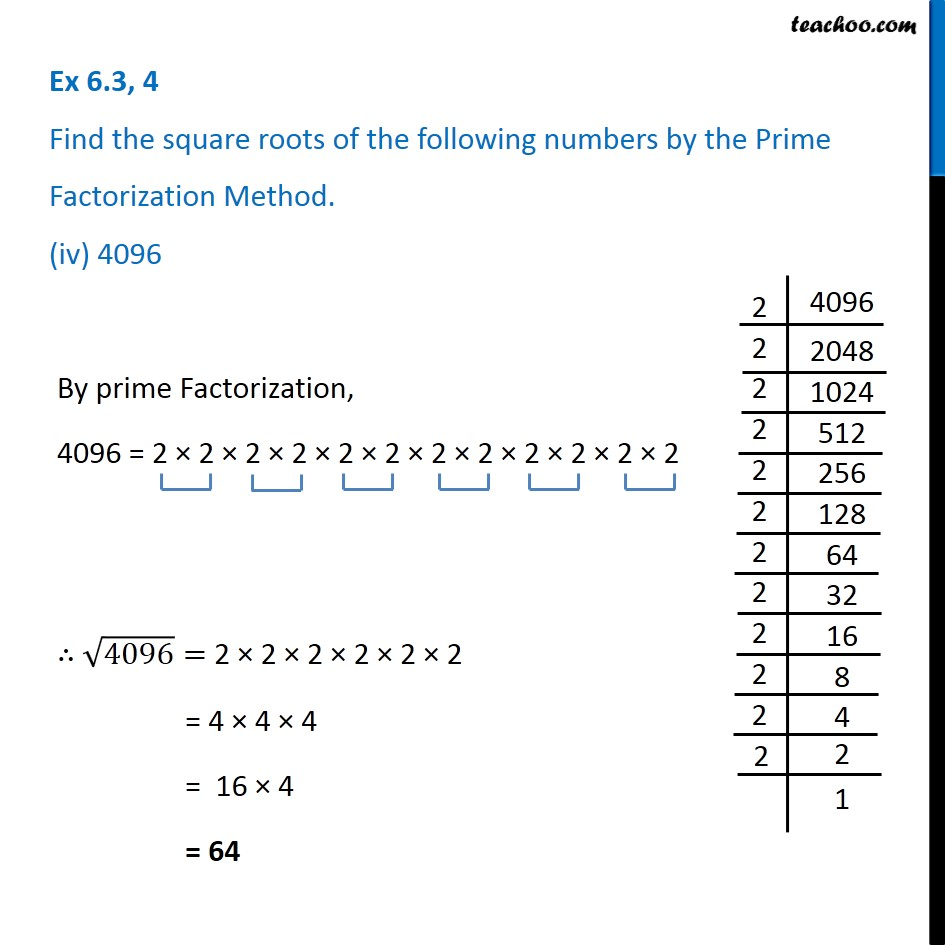Ex 6.3

Chapter 6 Class 8 Squares and Square Roots
Serial order wise### Transcript

Ex 6.3, 4 Find the square roots of the following numbers by the Prime Factorization Method. (iv) 4096 By prime Factorization, 4096 = 2 × 2 × 2 × 2 × 2 × 2 × 2 × 2 × 2 × 2 × 2 × 2 ∴ √4096= 2 × 2 × 2 × 2 × 2 × 2 = 4 × 4 × 4 = 16 × 4 = 64